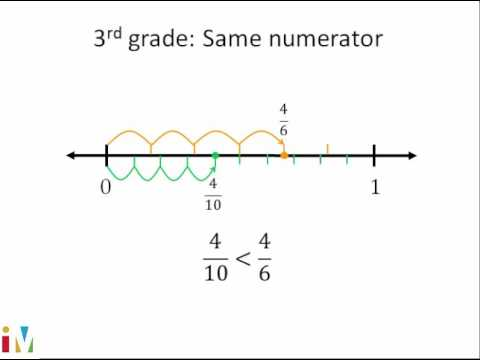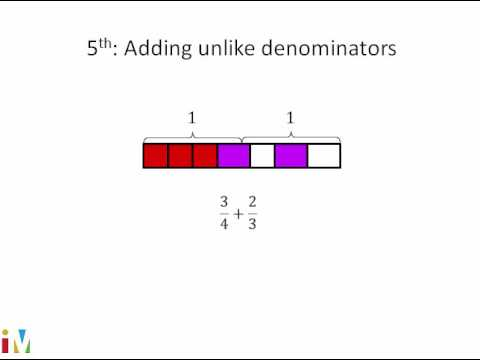Video

# Visualizing equivalent fractions (Full video)

Description: Sal uses fraction models to show equivalent fractions. Created by Sal Khan. Let's think about what fraction of this grid is actually shaded in pink. So the first thing we want to think about is how many equal sections do we have here? And how many of those equal sections are actually shaded in this kind of pinkish color?

### Other videos you might be interested in### Comparing Fractions

#### Illustrative Mathematics